# Program Using Expression in C++

## Program Using Expression in C++ with Examples:

In this article, I am going to discuss Program Using Expression in C++ with Examples. Please read our previous article where we discussed Operator Precedence and Expressions in C++ with Examples.

##### Program Using Expression in C++

Here, we will write a program for finding the area of a triangle. Now we will write a complete program, we already discussed what is the procedure in the previous article. Now we will see how to develop a program. So, every basic program will have three parts or three elements that are: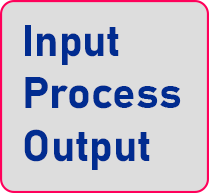It will take some input then process that and then show the output. So, these are the three steps that we will follow. So let us see for what we have to write on a program. We have to write a program for finding the area.

##### The formula of Area of Triangle is: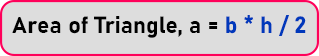In this program, input is: base and height and output is: Area of triangle. Now the program should take two inputs and then give the output.

##### Flow chart for Area of Triangle

So, for this first of all we will draw a flow chart: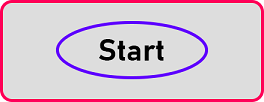So, this is starting of the flow chart. Next, we should take input.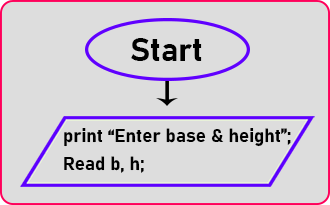So here print the message of “Enter base and height”. And then we will take variables b and h from the user. So, we read two values whatever a user gives. Here input part is over. Now, next is the formula. What is the formula? The formula of area: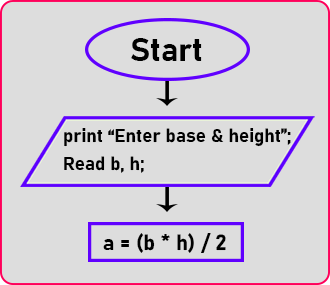Here process part is over. Now next is output. I have to give the result to the user. So here I should print the area. We will print the message “Area is” and then the area.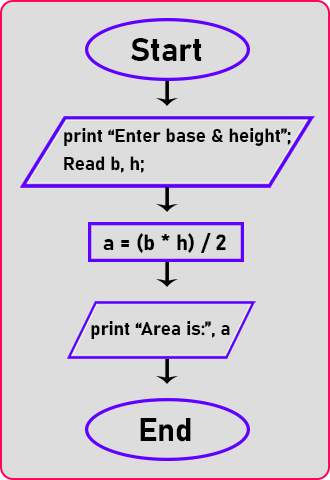I have the result in a so that’s all the output end of the flow chart. And here we have printed the output so this is the end of the flow chart.

##### Algorithm for Area of Triangle

And next thing I will write down the algorithm for this one. So, the algorithm will contain the same thing just I have to group all of them together. And after that, we will write a program. So, Algorithm is: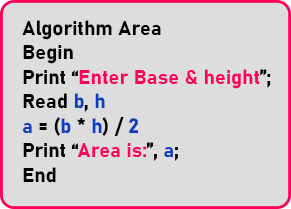We have written the same as the flowchart. So, there is no need to explain. Now we will write the program for C++:

##### Area of a Triangle Code in C++ Language:
```#include <iostream>
using namespace std;
int main ()
{
float base, height, area;
cout << "Enter Base & Height: ";
cin >> base >> height;
area = (base * height) / 2;
cout << "Area is: " << area;
return 0;
}
```
###### Output: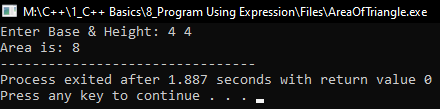In the next article, I am going to discuss the Sum of First N Natural Numbers in C++ with Examples. Here, in this article, I try to explain Program Using Expression in C++ with Examples and I hope you enjoy this Program Using Expression in C++ article.